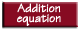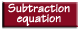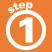Home    |    Teacher    |    Parents    |    Glossary    |    About UsTo solve an equation means to find a value for the variable that makes the equation true. Whatever you do to one side of the equation, you must also do to the other side.

Click the buttons to see how to solve each type of equation.Homework Help | Algebra | Equations and InequalitiesEmail this page to a friendSearch·  Solving addition and     subtraction equations·  Multiplication equations·  Division equations·  Inequalities·  Formulas·  Two-step equations     and inequalitiesFirst Glance In Depth Examples WorkoutSolving addition and subtraction equations